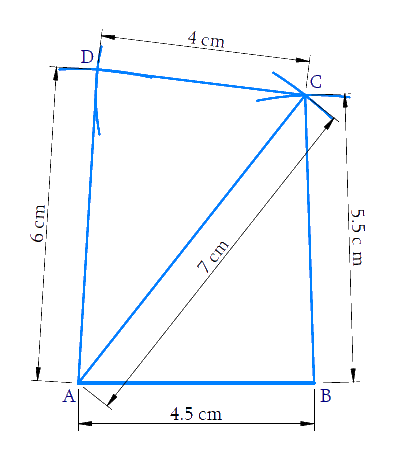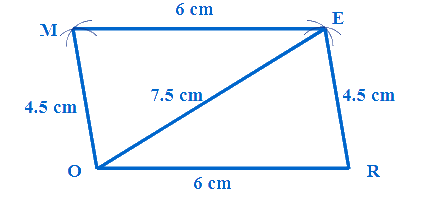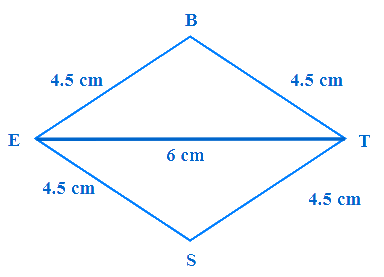# Excercise 4.1 Practical Geometry- NCERT Solutions Class 8

## Chapter 4 Ex.4.1 Question 1

Construct the following quadrilaterals.

(i) Quadrilateral $$ABCD.$$

$$AB = 4.5 \,\rm{cm}$$

$$BC = 5.5\,\rm{ cm}$$

$$CD = 4\,\rm{cm}$$

$$AD = 6\,\rm{cm}$$

$$AC = 7\,\rm{} cm$$

(ii) Quadrilateral $$JUMP$$

$$JU = 3.5\,\rm{cm}$$

$$UM = 4\,\rm{cm}$$

$$MP = 5\,\rm{cm}$$

$$PJ = 4.5\,\rm{cm}$$

$$PU = 6.5\,\rm{cm}$$

(iii) Parallelogram $$MORE$$

$$OR = 6\,\rm{cm}$$

$$RE = 4.5\,\rm{cm}$$

$$EO = 7.5\,\rm{cm}$$

(iv) Rhombus $$BEST$$

$$BE = 4.5\,\rm{cm}$$

$$ET = 6\,\rm{cm}$$

### Solution

What is the known?

Measurements of a Quadrilateral

What is unknown?

Construction of a Quadrilateral

Reasoning:

As you are aware, we need five measurements to draw a quadrilateral (Page 58 of Grade 8 NCERT Book)

Based on the given information, the five given measurements are of $$4$$ sides and one diagonal.When measurements of $$4$$ sides and one diagonal of a quadrilateral is given, we can construct a quadrilateral (Page 58 of Grade 8 NCERT Book)

Steps:

Let us first draw a rough sketch of quadrilateral.Construction of quadrilateral can be done in two parts. First construct triangle $$ABC$$ and then triangle $$ACD.$$ Let us find based on given measurements whether it is possible to construct the triangles.

In $$ABC,\;5.5+4.5>7$$ and $$5.5-4.5<7$$

$$7+4.5>5$$ and $$7-4.5<5.5$$

$$5.5+7>4.5$$ and $$7-5.5<4.5$$

It is possible to draw triangle $$ABC.$$

In $$ACD$$

$$7+4>6$$ and $$7-4<6$$

$$6+7>4$$ and $$7-6<4$$

$$6+4>7$$ and $$6-4<7$$

So construction of triangle $$ACD$$ is also possible.

Let us construct the quadrilateral.

Step 1: Draw line segment \begin{align}{{AB}} = 4.5\text{ cm}{{.}}\end{align} With $$B$$ as center and radius $$5.5\, \rm{cm}$$ draw an arc. With $$A$$ as center and radius $$7 \,\rm{cm}$$ draw another arc cutting the former arc. $$C$$ is the intersecting point of arcs. Join $$BC$$ and $$AC.$$

Step 2: Based on rough diagram, it is easy to find that $$AC$$ is the diagonal. Therefore, the fourth vertex $$D$$ should be on the opposite to $$B$$ with reference to $$AC$$.

Step 3: With $$A$$ as center and radius $$6\,\rm{cm}$$ draw an arc on the opposite side of point $$B.$$ With $$C$$ as center and radius $$4\,\rm{cm}$$ draw another arc cutting the former arc. $$D$$ is the intersecting point of the arcs. Join $$AD$$ and $$CD.$$ $$ABCD$$ is the required quadrilateral.

Related Problems.

Ex 4.1 (ii)

(iii) Parallelogram $$MORE$$

Using the concept opposite sides of parallelogram are parallel and equal, the five measurements can be found.(iv) Rhombus $$BEST$$

$$BE = 4.5\,\rm{cm}$$

$$ET = 6\, \rm{cm}$$

All sides of a rhombus are equal and opposite sides are parallel, measurements of all sides can be found.Instant doubt clearing with Cuemath Advanced Math Program